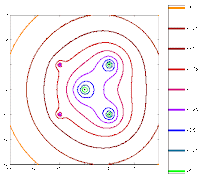## Page Views on Nuit Blanche since July 2010

My papers on ArXiv:
Approximating Kernels at the speed of Light
&
Imaging with Nature

LightOn
LinkedIn (727)|| on CrunchBase || our Blog
(2452)
(3967)
(1333)||
Attendant references pages:
The Advanced Matrix Factorization Jungle Page ||

Paris Machine Learning
@Meetup.com (8016 members) || @Archives

## Tuesday, July 03, 2007

### Random Projection Lapack ?Tamás Sarlós describes in this paper the use of random projections through the Fast JL transform so that one can reduce the size of very large matrices in order to compute their SVD. A related approach was taken by Thomas Strohmer and Roman Vershynin  to build a ranodmized solver with exponential convergence. It sounds like as if there is a potential for a Random Projection LAPACK (RP-LAPACK) but I wonder how these transformations will fare when confronted with Non-normal matrices or discretization of Non-normal operators. In this context, Non-normality has nothing to do with probability distributions but rather a way to describe Non hermitian matrices (or matrices/operators that do not commute with their transpose). In the case of high non-normality, these matrices have very wide spectral portraits. Corollary 4 of Sarlos' paper would point to the potential blow-up in the case of highly non-normal matrices, a situation not unlike the smashed filter case, where noisy data can overcome the tight bound on the projection in the lower dimension random space (theorem 1 of the smashed filter paper). High nonnormality occur whenever there exists a strong coupling between two or more phenomena, giving rise to high spectral instabilities. Examples include linear transport theory for neutrons but other applications where non-normality shows up are listed here. Using these matrices would help in figuring out how bad the problem is.

On a different note, Nick Trefethen pointed out that both some Random matrices and Toeplitz matrices have large pseudo-spectra:
The eigenvalues of finite banded Toeplitz matrices lie on curves in the complex plane that have been characterized by Schmidt and Spitzer [SS60]. In contrast, the spectrum of the corresponding infinite dimensional Toeplitz operator is the set of points in the complex plane that a(T) encloses with non-zero winding number; see Theorem 1.17 of [BS99]. The limit of the spectrum of banded Toeplitz matrices as the dimension goes to infinity is thus typically very different from the spectrum of the limit.

This uncomfortable situation can be resolved by considering the behavior of pseudospectra. Though the eigenvalues of the finite Toeplitz matrices may fall on curves in the complex plane, Landau [Lan75], Reichel and Trefethen [RT92b], and Böttcher [Böt94] have observed that the resolvent norm ||(zI-TN)-1|| grows exponentially in the matrix dimension for all z in the interior of the spectrum of the corresponding infinite dimensional operator. (As a result, it is difficult to accurately compute eigenvalues of non-symmetric banded Toeplitz matrices even for matrices of relatively modest dimensions, and this signals a warning against basing analysis of finite Toeplitz matrices based on eigenvalues alone.) Furthermore the above cited authors also concluded that the pseudospectra of TN converge to the pseudospectra of T.

Could Pseudo-spectra provide directions on successful implementation of compressive sampling using Toeplitz and Random matrices ? Does the fact that Random Matrices replacing Toeplitz matrices in compressed sensing help in thinking how one can devise a Deterministic Constructions of Compressed Sensing Matrices ? This would not be so outlandish since:

The Restricted Isometry Property is a condition on the spectral norm of the matrices A^T = Phi* Phi
[ Update: Terry Tao just wrote to explain the issue with building deterministic UUP matrices ]

On a totally different note, I just found this paper on Identification of Matrices having a Sparse Representation by Holger Rauhut, Gotz Pfander and Jared Tanner. Initially derived for wireless communication inverse problem and sonar, it could readily be applied to neutron transport as well.

Reference:
 T. Strohmer, R. Vershynin, A randomized solver for linear systems with exponential convergence, RANDOM 2006 (10th International Workshop on Randomization and Computation), Springer Lecture Notes in Computer Science 4110, 499--507. Journal version: A randomized Kaczmarz algorithm with exponential convergence, Journal of Fourier Analysis and Applications, to appear